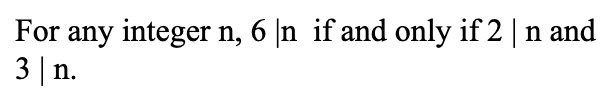# For any integer n, 6 n if and only if 2 | n and 3|n.

Question-AnswerCategory: MathematicsFor any integer n, 6 n if and only if 2 | n and 3|n.

For any integer n, 6 n if and only if 2 | n and 3|n.Given that,
For any integer n, 6|n if and only if 2|n and 3|n.
6|n is means n is divisible by 6.
So from the given statement we need to prove, if n is divisible by 6 if and only if n is divisibly by 2 and n is divisible by 3.
we know that 6 = 2 * 3
6k = (2*3)k where k = 1, 2, 3, 4, ….

So anything is multiple of 6 is divisible by both 2 and 3.

which means if n is divisible by 6 then it will definitely divisible by both 2 and 3.

Let assume n is divisible by both 2 and 3.
So n = 2m * 3r
n = 6 * m * r

So n is also divisible by 6.

Hence proved.